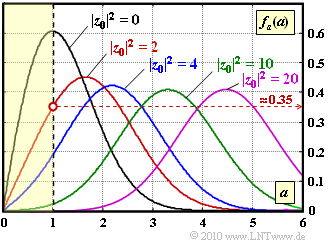# Exercise 1.7: PDF of Rice FadingRice fading for different values of  $|z_0|^2$

As you can see in the diagram, we consider the same scenario as in  Exercise 1.6:

• Rice fading  with variance of the Gaussian processes   $\sigma^2 = 1$  and parameter  $|z_0|$  for the direct path.
• Regarding direct path, we are interested in the parameter values  $|z_0|^2 = 0, \ 2, \ 4, \ 10, \ 20$  (see graph).
• The PDF of the magnitude  $a(t) = |z(t)|$  is
$$f_a(a) ={a}/{\sigma^2} \cdot {\rm e}^{ -{(a^2+ |z_0|^2) }/({2\sigma^2})}\cdot {\rm I}_0 \left [ {a \cdot |z_0|}/{\sigma^2} \right ]\hspace{0.05cm}.$$
• For example, the modified zeroth order Bessel function returns the following values:
$${\rm I }_0 (2) = 2.28\hspace{0.05cm},\hspace{0.2cm}{\rm I }_0 (4) = 11.30\hspace{0.05cm},\hspace{0.2cm}{\rm I }_0 (3) = 67.23 \hspace{0.05cm}.$$
• The power (noncentral second moment) of the multiplicative factor  $|z(t)|$ is
$${\rm E}\left [ a^2 \right ] = {\rm E}\left [ |z(t)|^2 \right ] = 2 \cdot \sigma^2 + |z_0|^2 \hspace{0.05cm}.$$
• With  $z_0 = 0$,  the Rice fading  becomes Rayleigh fading, which is more critical.  In this case, the probability that  $a$  lies in the yellow-shaded area between  $0$  and  $1$  is
$${\rm Pr}(a \le 1) = 1 - {\rm e}^{-0.5/\sigma^2} \approx 0.4 \hspace{0.05cm}.$$

In this task the probability  ${\rm Pr}(a ≤ 1)$  for  $|z_0| ≠ 0$  is to be approximated. There are two ways to do this, namely:

• the triangular approximation:   ${\rm Pr}(a \le 1) = {1}/{2} \cdot f_a(a=1) \hspace{0.05cm}.$
• the Gaussian approximation:   If   $|z_0| \gg \sigma$, then the Rice distribution can be approximated by a Gaussian distribution with mean  $|z_0|$  and standard deviation  $\sigma$ .

Notes:

### Questions

1

Calculate some PDF values for  $|z_0| = 2$  and  $\sigma = 2$:

 $f_a(a = 1) \ = \$ $f_a(a = 2) \ = \$ $f_a(a = 3) \ = \$

2

Let   $|z_0| = 2$   ⇒   $|z_0|^2 = 4$  (blue curve).  How big is  ${\rm Pr}(a ≤ 1)$?  Use the  triangular approximation.

 ${\rm Pr}(a ≤ 1)\ = \$ $\ \%$

3

Let   $|z_0|^2 = 2$  (red curve).  How big is  ${\rm Pr}(a ≤ 1)$?  Use the  triangular approximation.

 ${\rm Pr}(a ≤ 1) \ = \$ $\ \%$

4

Let  $|z_0|^2 = 10$  (green curve).  How big is  ${\rm Pr}(a ≤ 1)$?  Use the  Gaussian approximation.

 ${\rm Pr}(a ≤ 1) \ = \$ $\ \%$

5

Let  $|z_0|^2 = 20$  (purple curve).  How big is  ${\rm Pr}(a ≤ 1)$?  Use the  Gaussian approximation.

 ${\rm Pr}(a ≤ 1) \ = \$ $\ \%$

### Solution

#### Solution

(1)  With  $|z_0| = 2$  and  $\sigma = 2$  the Rice PDF is

$$f_a(a) = a \cdot {\rm exp} [ -\frac{a^2 + 4}{2}] \cdot {\rm I}_0 (2a)\hspace{0.05cm}.$$
• This gives the desired values:
$$f_a(a = 1) \hspace{-0.1cm} \ = \ \hspace{-0.1cm} 1 \cdot {\rm e}^{-2.5} \cdot {\rm I}_0 (2) = 0.082 \cdot 2.28 \hspace{0.15cm} \underline{ = 0.187}\hspace{0.05cm},$$
$$f_a(a = 2) \hspace{-0.1cm} \ = \ \hspace{-0.1cm} 2 \cdot {\rm e}^{-4} \cdot {\rm I}_0 (4) = 2 \cdot 0.0183 \cdot 11.3 \hspace{0.15cm} \underline{ = 0.414}\hspace{0.05cm},$$
$$f_a(a = 3) \hspace{-0.1cm} \ = \ \hspace{-0.1cm} 3 \cdot {\rm e}^{-6.5} \cdot {\rm I}_0 (6) = 3 \cdot 0.0015 \cdot 67.23 \hspace{0.15cm} \underline{ = 0.303}\hspace{0.05cm}.$$
• The results fit well with the blue curve on the graph.

(2)  With the result of the subtask  (1)   ⇒   $f_a(a = 1) = 0.187$  the triangle approximation gives

$${\rm Pr}(a \le 1) = {1}/{2} \cdot 0.187 \cdot 1\hspace{0.15cm} \underline{ \approx 9.4\,\%} \hspace{0.05cm}.$$
• This result will be a bit too large, because the blue curve is below the connecting line from  $(0, 0)$  to  $(1, 0.187)$   ⇒   convex curve.

(3)  For the red curve the PDF value  $f_a(a = 1) \approx 0.35$  can be read from the graph:

$${\rm Pr}(a \le 1) = \frac{1}{2} \cdot 0.35 \hspace{0.15cm} \underline{ \approx 17.5\,\%} \hspace{0.05cm}.$$
• The actual probability value will be slightly larger because the red curve is concave in the range between  $0$  and  $1$.

(4)  The Gaussian approximation states that one can approximate the Rice distribution by a Gaussian distribution with mean  $|z_0| = \sqrt{10} = 3.16$  and standard deviation  $\sigma = 1$  if the quotient  $|z_0|/\sigma$   is sufficiently large.  Then we have

$${\rm Pr}(a \le 1) \approx {\rm Pr}(g \le -2.16) = {\rm Q}(2.16) \hspace{0.15cm} \underline{ \approx 1.5\,\%} \hspace{0.05cm}.$$
• Here,  $g$  denotes a Gaussian distributed random variable with mean  $0$  and standard deviation  $\sigma = 1$.
• The numerical value was determined with the specified interactive applet  Complementary Gaussian Error Functions.

Note:   The Gaussian approximation is certainly associated with a certain error here:

• From the graph you can see that the average value of the green curve is not  $a = 3.16$,  but rather  $3.31$.
• Then the power of the Gaussian approximation  $(3.31^2 + 1^2 = 12)$  is exactly the same as that of the Rice distribution:
$$|z_0|^2 + 2 \sigma^2= 10 + 2 =12\hspace{0.05cm}.$$

(5)  Using the same calculation method, replace the Rice PDF with a Gaussian PDF with mean value  $\sqrt{20} \approx 4.47$  and standard deviation  $\sigma = 1$  and you get

$${\rm Pr}(a \le 1) \approx {\rm Pr}(g \le -3.37) = {\rm Q}(3.37) { \approx 0.04\,\%} \hspace{0.05cm}.$$
• If one assumes the equal power Gaussian distribution  (see the note to the last subtask), the mean value is  $m_g = \sqrt{21}\approx 4.58$,  and the probability would then be
$${\rm Pr}(a \le 1) \approx {\rm Q}(3.58) \hspace{0.15cm} \underline{ \approx 0.02\,\%} \hspace{0.05cm}.$$